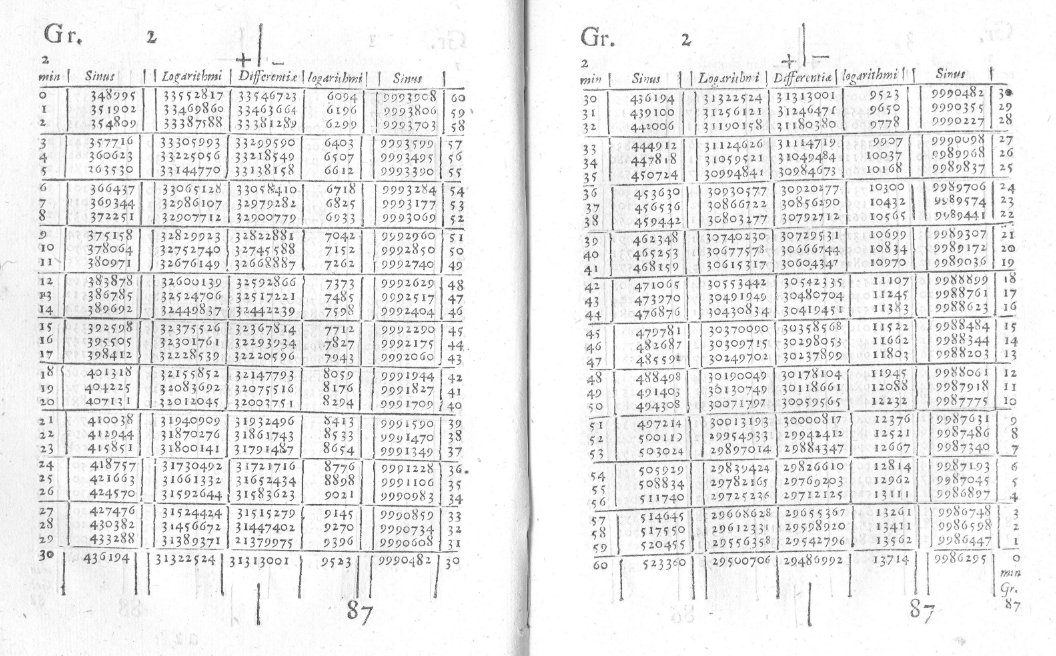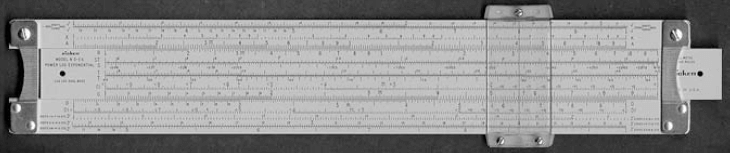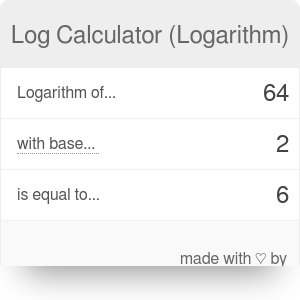Logarithm of...
with base...
... equals

# Log Calculator (Logarithm)

By Bogna Haponiuk and Tibor Pal, PhD candidate

This log calculator (logarithm calculator) allows you to calculate the logarithm of a (positive real) number with a chosen base (positive, not equal to 1). Regardless of whether you are looking for a natural logarithm, log base 2, or log base 10, this tool will solve your problem. Read on to get a better understanding of the logarithm formula and the rules you need to follow. Besides, you might find some fascinating information, such as why logarithm are so essential in our life and where they are applied.

If you are searching also for other useful maths calculators do not hesitate to take a look at our cube root calculator which enables you to calculate not only the cube root but also roots to any degree.

## What is a logarithm?

A logarithmic function is an inverse of the exponential function. In essence, if a raised to power y gives x, then the logarithm of x with base a is equal to y. In the form of equations,

`aʸ = x` is equivalent to `logₐ(x) = y`.

In other words, the logarithm of x, or logₐ(x), shows what power we need to raise a to (or if x is greater than 1, how many times a needs to be multiplied by itself) to produce the value x. From this view, we can represent the logarithm in the following way as well:

`aˡᵒᵍₐ⁽ˣ⁾ = x`

Hopefully you now understand the definition of logarithm; in the following section you can read about the two most frequently used forms of it.

## The natural logarithm and the common logarithm

You can choose various numbers as the base for logarithms; however, there are two particular bases that are used so often that mathematicians have given unique names for them, the natural logarithm and the common logarithm.

• Natural logarithm

If you want to compute the natural logarithm of a number, you need to choose a base that is approximately equal to 2.718281. Conventionally this number is symbolized by e, named after Leonard Euler who defined its value in 1731. Accordingly, the logarithm can be represented as logₑx, but traditionally it is denoted with the symbol ln(x). You might also see log(x) which also refers to the same function, especially in finance and economics. Therefore,

`y = logₑx = ln(x)` which is equivalent to `x = eʸ = exp(y)`.

One practical way to understand the function of the natural logarithm is to put in the context of compound interest, that is, the interest that is calculated on both the principal and the accumulated interest.

The formula for annual compound interest is as follows:

`A = P * (1 + r/m)ᵐᵗ`

Where:

• A is the value of the investment after t years
• P stands for the initial value
• r is the annual interest rate (in decimals)
• m represents the number of times the interest is compounded per year or compounding frequency
• t refers to the numbers of years

Let's assume that you deposit some money for a year in a bank where compounding frequently occurs, thus m equal to a large number. It is easy to see how quickly the value of m is increasing if you compare yearly (m=1), monthly (m=12), daily (m=365), or hourly (m=8,760) frequencies. Now, let's imagine that your money is recalculated in every minutes or second: the m became a considerably high number.

Now let's check how the growing frequency affects your initial money:

CSV To HTML using codebeautify.org
m (1 + r/m)ᵐ
1 2
10 2.59374…
100 2.70481…
1000 2.71692…
10,000 2.71814…
100,000 2.71826…
1,000,000 2.71828…

You may notice that even though the frequency of compounding reaches an unusually high number, the value of `(1 + r/m)ᵐ` (which is the multiplier of your initial deposit) doesn't increase very much. Instead, it becomes somewhat stable: it's approaching a unique value already mentioned above, `e ≈ 2.718281`.

Since growth rates often follow a similar pattern as the above example, economics also heavily rely on natural logarithm. Two common variables involve natural logarithm: the GDP growth rate and the price elasticity of demand.

• Common logarithm

The other popular form of a logarithm is the common logarithm with the base of 10, log₁₀x, which is conventionally denoted as lg(x). It is also known as the decimal logarithm, the decadic logarithm, the standard logarithm or the Briggsian logarithm, named after Henry Briggs, an English mathematician who developed its use.

As its name suggests, it is the most frequently used form of logarithm. It is used, for example, in our decibel calculator. Logarithm tables that aimed at easing computation in the olden times usually presented common logarithms, too.

The below table represents some frequent number common and natural logarithms.

x log₁₀x logₑx
0 undefined undefined
0+ - ∞ - ∞
0.0001 -4 -9.21034
0.001 -3 -6.907755
0.01 -2 -4.60517
0.1 -1 -2.302585
1 0 0
2 0.30103 0.693147
3 0.477121 1.098612
4 0.60206 1.386294
5 0.69897 1.609438
6 0.778151 1.791759
7 0.845098 1.94591
8 0.90309 2.079442
9 0.954243 2.197225
10 1 2.302585
20 1.30103 2.995732
30 1.477121 3.401197
40 1.60206 3.688879
50 1.69897 3.912023
60 1.778151 4.094345
70 1.845098 4.248495
80 1.90309 4.382027
90 1.954243 4.49981
100 2 4.60517
200 2.30103 5.298317
300 2.477121 5.703782
400 2.60206 5.991465
500 2.69897 6.214608
600 2.778151 6.39693
700 2.845098 6.55108
800 2.90309 6.684612
900 2.954243 6.802395
1000 3 6.907755
10000 4 9.21034

## How to calculate logarithm with arbitrary base

If you want to compute a logarithm with an arbitrary base, but can access only a natural logarithm calculator or a log 10 base calculator, you need to apply the following rules:

`logₐ(x) = ln(x) / ln(a)`

`logₐ(x) = lg(x) / lg(a)`

## Log base 2: an example

Let's assume you want to use this tool as a log base 2 calculator. To calculate the logarithm of any number, simply follow these simple steps:

1. Decide on the number you want to find the logarithm of. Let's say it's 100.
2. Decide on your base - in this case, 2.
3. Find the logarithm with base 10 of number 100. `lg(100) = 2`.
4. Find the logarithm with base 10 of number 2. `lg(2) = 0.30103`.
5. Divide these values by one another: `lg(100)/lg(2) = 2 / 0.30103 = 6.644`.
6. You can also skip steps 3-5 and input the number and base directly into the log calculator.

## History of the logarithm

Evidence suggests that the notion of logarithms was already present in 8th century India. However, the developed concept of logarithm appeared first time in the book titled Mirifici logarithmorum canonis descriptio (The construction of the marvelous canon of logarithms) printed in 1614. It was the result of a 20 year's of research by the Scottish mathematician, John Napier, aimed to ease computation applied for his other preoccupation as an astronomer and physicist.Source: Havil, Julian (2014). John Napier. Life, logarithms, and legacy. Princeton University Press

The first part of the book precisely explains the theory of the logarithm with its properties and application areas. The second part of the book illustrates examples of logarithmic computations through ninety pages of tables. Napier also enunciates the limitations of his work and provides analogies, examples, warnings, reminders, and conclusions. Therefore the book can function as an instruction manual. He doesn't, however, elaborate on how the tool was created.The word logarithm is derived from a composition of two Greek words: logos, which refers to a ratio, and arithmos, which has the meaning number. Therefore, logarithms are ratio numbers; numbers which relate to ratios.

For our generation, it might be difficult at first glance to appreciate the invention of the logarithm since we already employ modern calculators and computers for mathematical computations. However, back in the 17th century, it was a canonical discovery that had a profound impact on people's lives. To resolve mathematical problems before the appearance of logarithms could take hours, days, or even years. The first explicit advancement that the logarithms brought forward was the enhancement of computations by converting multiplication and divisions into addition and subtraction. The only additional effort was the looking up logarithms and antilogarithms in tables.

The new computational procedure was instrumental in the field of astronomy. Napier's scientific activities coincided with the era of new developments in astrophysics. As a result, many astronomers were striving with endless calculations aimed at detecting the position of the planets using Copernicus's theory of the solar system. Johannes Kepler, at the time working on his famous laws of planetary motions, was among them. Thanks to Napier's endeavor, he could reduce his workload substantially which previously demanded close to a thousand pages of computations, allowing him to devote more time to philosophical speculations.

The famous British mathematician, Henry Briggs, quickly realized the capability of the new invention: he moved to Scotland to meet Napier so they could begin to search for potential advancements together. As a result, after modifying the original idea, in 1617, they formulated the first table of the logarithms based on powers of 10. In 1624, after Napier's death, Briggs published his book, Arithmetica logarithmic that presented logarithmic tables for 30 thousand natural numbers to 14 decimal places. This form of logarithms is considered today as common logarithms. (Katrici, 2007)

## The slide rules

The growing popularity of the new mathematical instrument stimulated further explorations. In 1620 Edmund Gunter introduced the calculating line of the logarithm, a physical device used for multiplications and divisions. The early version of the tool, which required a pair of compasses for measuring, was advanced by William Oughtred around 1622. He designed the conventional slide rule, the device which has two rulers sliding next to each other.In this way, he created a new approach for the further easing of computing the law of logarithm. Slide rule became a common computational device in professions demanding arithmetic. Architects, engineers, scientists and even astronauts were relying on its calculative aid until the advent of the Digital Revolution. Albert Einstein was using it, and the crews of the Apollo missions also took slides rules to space. Compared to the early version of computers, slide rule had many advantages:

• small enough to keep in a pocket,
• doesn't require a power source,
• relatively cheap,
• mechanically reliable,
• simple to function,
• can solve any numerical problem related to regular conditions.## Logarithms in the real world

In the present day, modern computers and scientific calculators replaced the old-fashioned practices. Still, understanding the concepts behind logarithms can help you to develop your mathematical skills. Logarithms still have multiple practical uses in many fields.

The fact that logarithms relate arithmetic progression to geometric progression suggests that certain phenomena in the real world might form a logarithmic pattern. Indeed, there are an abundant example in nature and our practical life which can be attributed to the magical logarithm.

For example, the following natural phenomena depict a logarithmic spiral:

• shell of a nautilus• galaxies• cyclonesBesides, there are other phenomena which are measured on the logarithmic scale:

• mineral hardness - Mohs Scale,
• intensities of sounds - Decibels (dB),
• wind intensity - Beaufort Scale,
• earthquakes - Richter Scale,
• acidity - pH.

## Applying logarithm for arithmetic computations

Before the end of the 70's, when pocket calculators became accessible for the regular public, carrying out computations, especially with fractions, demanded a substantial manual effort. To alleviate the tedious work, the application of logarithms served a practical function.

For utilizing the technical advantage of the logarithm we need to be familiar with its basic properties. Presumably, you already met these rules but, to remind you, the table below displays them.

Rule or special case Formula
Product ln(x*y)=ln(x)+ln(y)
Quotient ln(x/y)=ln(x)−ln(y)
Log of power ln(xy)=y*ln(x)
Log of e ln(e)=1
Log of one ln(1)=0
Log reciprocal ln(1/x)=−ln(x)

To demonstrate how useful it was in pre-calculator times, let's assume that you need to compute the product of `5.89 * 4.73` without any electronic device. You could do it by merely multiplying things out on paper; however, it would take a bit of time. Instead, you can use the rule of logarithm with log tables and get a relatively good approximation of the result.

If you had a log table, you could quickly check the logarithm of these numbers (or you use the Internet to find an electronically uploaded table), but let's cheat a bit and use our calculator for this purpose now.

`lg(5.89) ≅ 0.7701153` and `lg(4.73) ≅ 0.674861`

By applying the first rule we can rewrite the following equation:

`lg(5.89*4.73) = ln(5.89) + lg(4.73) ≅ 0.770115 + 0.6748611`

`lg(5.89*4.73) ≅ 1.4449761`

We still don't know what the exact result is, so we take the exponent of both side of the equation above with some change on the right side.

`5.89*4.73 ≅ 10¹·⁴⁴⁴⁹⁷⁶¹ = 10⁰·⁴⁴⁴⁹⁷⁶¹ * 10¹`

Now what you would need to do is to check 100.4449761 in an anti-log table or alternatively you check in our antilog calculator. What is the antilog of `0.4449761` in base 10? `2.785968`.

Rewriting the equation:

`5.89*4.73 ≅ 2.785968 * 10¹ = 27.85968`

Most probably, the above procedure seems to be demanding compared to just entering it into a pocket calculator or using any capable application like our calculator. To show how the power of logarithm might help you out even in our modern times, let's consider a factorial operation of 100 which is the product of all integers from 1 to 100.

`100! = 1 * 2 * 3 * 4 * ⋯ * 99 * 100`

If you were trying to resolve this problem by a regular pocket calculator you would probably fail as a result is a huge number with copious digits.

But with the help of algorithm you may rewrite (with some rounding) the operation as:

`ln100!=lg1 + lg2 + lg3 + ⋯ + lg100 = 0 + 0.30103 + 0.47712 + ⋯ + 2 ≅ 157.97`

`100! ≅ 10⁰·⁹⁷ * 10¹⁵⁷ = 9.3325 * 10¹⁵⁷`

• http://astro.wsu.edu/hclee/APOD.html
• http://abitofauldmaths.org
• http://www.montereyinstitute.org
• Havil, Julian (2014). John Napier. Life, logarithms, and legacy. Princeton University Press

## Get the widget!

Log Calculator (Logarithm) can be embedded on your website to enrich the content you wrote and make it easier for your visitors to understand your message.

It is free, awesome and will keep people coming back!